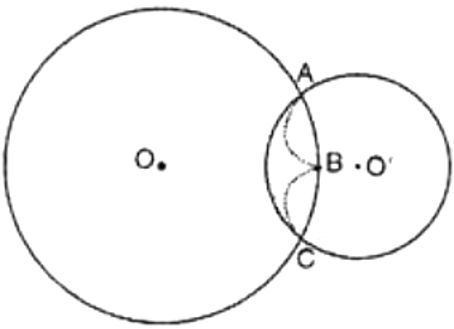No products in the cart.

# Draw different pairs of circle how …## CBSE, JEE, NEET, CUET

Question Bank, Mock Tests, Exam Papers

NCERT Solutions, Sample Papers, Notes, Videos

Draw different pairs of circle how many points does each pair have in common what is the maximum number of common points

Preeti Dabral 1 month, 3 weeks ago

From the figure, we observe that when different pairs of circles are drawn, each pair have two points (say A and B) in common.
Maximum number of common points are two in number.Suppose two circles C (O) and C (O’) intersect each other in three points, say A, B and C.
Then A, B and C are non-collinear points.
We know that:
There is one and only one circle passing through three non-collinear points.
Therefore, a unique circle passes through A, B and C.
{tex} \Rightarrow {/tex} O’ coincides with O and s = r where s and r are the radii of two circles C(O) and C(O')
A contradiction to the fact that C (O’,) {tex} \ne {/tex} C (O,)
{tex}\therefore {/tex} Our supposition is wrong.
Hence two different circles cannot intersect each other at more than two points.

Related Questions## myCBSEguide

Trusted by 1 Crore+ Students

#### Question Paper Creator

• Create papers in minutes
• Print with your name & Logo
• 5 Lakhs+ Questions
• Solutions Included
• Based on CBSE Syllabus
• Best fit for Schools & Tutors## Test Generator

Create papers at ₹10/- per paper## CUET Mock Tests

75,000+ questions to practice only on myCBSEguide app